﻿ NRectangle Structure Members
Nevron .NET Vision
Nevron.GraphicsCore Namespace / NRectangle Structure
Fields Properties Methods

In This Topic
NRectangle Structure Members
In This Topic

The following tables list the members exposed by NRectangle.

Public Constructors
NameDescription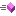Overloaded.
Top
Public Fields
NameDescription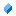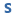The empty rectangleRectangle heightRectangle widthX coordinate of the left top cornerY coordinate of the left top corner
Top
Public Properties
NameDescription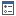Gets the minimal enclosing rectangleGets the minimal enclosing 2D rangeDetermines whether this is a flat primitive
Top
Public Methods
NameDescriptionAppends the primitive to the specified graphics pathDetermines whether the area of this rectangle intersects with the area of the specified circleOverloaded. Determines whether the rectangle contains the specified pointOverloaded. Determines whether the outline of the rectangle contains the specified pointReturns the fitted rectangle with the specified orientation (in degrees)Gets a rectangle from center and dimensionsGets a rectangle from the coordinates of it's sidesOverloaded. Gets a normalized rectangle from two pointsObtains the point on the rectangle rim, which corresponds to the specified compass directionObtains the point on the rectangle rim, which corresponds to the specified compass directionIncludes the point in this rectangleDetermines whether the rectangle contains the point with the specified coordinatesDetermines whether this rectangle intersects with the specified rectangleOverloaded. Inflates the rectangle with the specified amountsReturns a third rectangle that represents the intersection of two rectangles. If there is no intersection, the Empty rectangle is returnedOverloaded. Determines whether this rectangle intersects with the specified rectangleIntersects this rectangle with the specified line segmentIntersects this rectangle with a line connecting the rectangle center and the specified pointIntersects this rectangle with the ray from the bounds central pointNormalizes this rectangleOffsets the rectangle with the specified x and y amount.Computes a new rect for an expected resize of a rectangle in the specified compass directionScales the rectangle with the specified factorsConverts this rectangle to NRange2DFConverts this rectangle to RectangleFConverts this rectangle to RectangleConverts this rectangle to RectangleFReturns the string representation of this objectOverloaded. Translates the primitive with the specified amountsCreates the smallest possible third rectangle that can contain the two rectangles that form a union
Top
Public Operators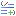Equality Determines whether a is equal to bInequality Determines whether a is not equal to b
Top